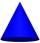# Body volume of a cone problems

#### Number of problems found: 69

• Cut and coneCalculate the volume of the rotation cone which lateral surface is circle arc with radius 15 cm and central angle 63 degrees.
• The cone - S,VCalculate the volume and surface area of the cone if its radius r = 6 cm and side s = 10 cm.
• Cone area and sideCalculate the surface area and volume of a rotating cone with a height of 1.25 dm and 17,8dm side.
• Volume of the coneFind the volume of the cone with the base radius r and the height v. a) r = 6 cm, v = 8 cm b) r = 0,9 m, v = 2,3 m c) r = 1,4 dm, v = 30 dm
• Volume of the coneCalculate the volume of the cone if the content of its base is 78.5 cm2 and the content of the shell is 219.8 cm2.
• The diagram 2The diagram shows a cone with slant height 10.5cm. If the curved surface area of the cone is 115.5 cm2. Calculate correct to 3 significant figures: *Base Radius *Height *Volume of the cone
• ConeCalculate volume and surface area of ​​the cone with a diameter of the base d=15 cm and side of the cone with the base has angle 52°.
• ConeThe rotating cone volume is 9.42 cm3, with a height 10 cm. What angle is between the side of the cone and its base?
• Rotating coneCalculate the volume and the surface area of a rotating cone of base radius r = 2.3 dm and a height h = 46 mm.
• Max - coneFrom the iron bar (shape = prism) with dimensions 6.2 cm, 10 cm, 6.2 cm must be produced the greatest cone. a) Calculate cone volume. b) Calculate the waste.
• Cone - sideFind the surface area and volume of the cone if its height is 125 mm and the side length is 17 cm.
• Slant heightThe cone's slant height is 5cm, and the radius of its base is 3cm, find the volume of the cone.
• Cone containerRotary cone-shaped container has a volume 1000 cubic cm and a height 12 cm. Calculate how much metal we need for making this package.
• Truncated cone 6Calculate the volume of the truncated cone whose bases consist of an inscribed circle and a circle circumscribed to the opposite sides of the cube with the edge length a=1.
• Axial sectionThe axial section of the cone is an equilateral triangle with area 168 cm2. Calculate the volume of the cone.
• Rotating coneFind the surface and volume of the rotating cone if its side is 150 mm long and the circumference of the base is 43.96 cm.
• Truncated coneA truncated cone has a bases radiuses 40 cm and 10 cm and a height of 25 cm. Calculate its surface area and volume.
• Surface areaThe volume of a cone is 1000 cm3 and the content area of the axis cut is 100 cm2. Calculate the surface area of the cone.
• Ice cream in coneIn the ice cream cone with a diameter of 5.7 cm is 0.8 dl of ice cream. Calculate the depth of the cone.
• Axial section of the coneThe axial section of the cone is an isosceles triangle in which the ratio of cone diameter to cone side is 2: 3. Calculate its volume if you know its area is 314 cm square.

Do you have an interesting mathematical word problem that you can't solve it? Submit a math problem, and we can try to solve it.

We will send a solution to your e-mail address. Solved examples are also published here. Please enter the e-mail correctly and check whether you don't have a full mailbox.

Please do not submit problems from current active competitions such as Mathematical Olympiad, correspondence seminars etc...